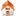# Quantitative characterization of coal by means of microfocal X-ray Computed Microtomography (CMT) and Color Image Analysis (CIA)

## Frederik J Simons, Frédéric Verhelst and Rudy Swennen

### Fysico-Chemische Geologie & Department of Civil Engineering Katholieke Universiteit Leuven (KUL)Leuven, Belgium

Int. J. Coal Geol. 34, 69-88, 1997.

 Reprint Related work Publications

#### Figures

1. Figure 01 Energy dependence of the linear attenuation coefficient for hexagonal carbon
2. Figure 02 The microfocal X-ray tube
3. Figure 03 Geometrical unsharpness
4. Figure 04 Reduction of intrinsic unsharpness
5. Figure 05 Schematic representation of Color Image Analysis (CIA)
6. Figure 06 Photograph of the surface of the coal sample
7. Figure 07 Computerized Micro Tomography tomodensities of the coal sample
8. Figure 08 Cumulative normalized surface percentages of the five constituents (vitrinite, liptinite, inertinite, pyrite and binder) sampled along trace 1
9. Figure 09 Cumulative normalized surface percentages of the five constituents (vitrinite, liptinite, inertinite, pyrite and binder) sampled along trace 2
10. Figure 10 Filtered normalized surface percentages of trace 1
11. Figure 11 Filtered normalized surface percentages of trace 2
12. Figure 12 Results of the multiple regression for trace 1: predicted versus observed tomodensity
13. Figure 13 Results of the multiple regression for trace 2: predicted versus observed tomodensity
14. Figure 14 Predicted versus observed tomodensity after breakpoint regression
15. Figure 15 Experimental semivariogram for the virtinite surface percentage of trace 1
16. Figure 16 Experimental semivariogram for the virtinite surface percentage of trace 2

Frederik Simons